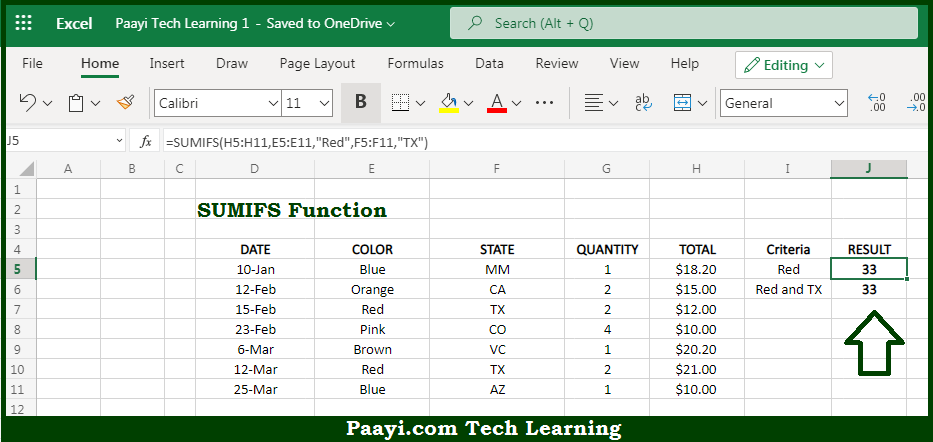# Learn How to Use Microsoft Excel SUMIFS Function

Written by | 0 Comments | 578 Views

In this article, you will learn how to use the Microsoft Excel SUMIFS function and its prime function in Microsoft Excel. You will also get to know the Microsoft Excel SUMIFS function return value and syntax with the help of some examples.

Microsoft Excel SUMIFS Function

The main function of the Microsoft Excel SUMIFS function is to sum the cells that match multiple criteria. That implies, with the help of the SUMIFS function you can able to return the sum of cells that meet multiple conditions. It should be noted that SUMIFS functions can be used to sum values when adjacent cells meet criteria based on dates, numbers, and text. The SUMIFS function also supports logical operators like (>,<,<>,=) and wildcards (*,?) for partial matching. So, with the help of the SUMIFS function, you can able to sum the cells that match multiple criteria.

Return Value of SUMIFS Function

The return value will be the sum of cells that meet multiple criteria.

Syntax of SUMIFS Function

=SUMIFS(sum-range, range1, criteria1, [range2], [criteria2], ...)

Where the arguments:

• sum-range: This is the range to be summed.
• range1: This is the first range to evaluate.
• criteria1 This is the criteria to use on range1.
• range2: This is the second range to evaluate (optional).
• criteria2: This is the criteria to use on range2 (optional).

## How to Use Microsoft Excel SUMIFS Function?So we know that Microsoft Excel SUMIFS function you can able to sum the cells that match multiple criteria. That implies, with the help of the SUMIFS function you can able to return the sum of cells that meet multiple conditions. It should be noted that SUMIFS functions can be used to sum values when adjacent cells meet criteria based on dates, numbers, and text. The SUMIFS function also supports logical operators like (>,<,<>,=) and wildcards (*,?) for partial matching. So, with the help of the SUMIFS function, you can able to sum the cells that match multiple criteria.